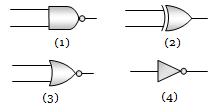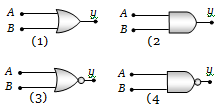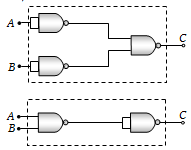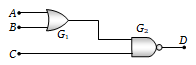Given below are symbols for some logic gatesThe XOR gate and NOR gate respectively are
(a) 1 and 2                  (b) 2 and 3
(c) 3 and 4                  (d) 1 and 4

Concept Questions :-

Logic gates
High Yielding Test Series + Question Bank - NEET 2020

Difficulty Level:

Given below are four logic gate symbol (figure). Those for OR, NOR and NAND are respectively(a) 1, 4, 3                     (b) 4, 1, 2
(c) 1, 3, 4                     (d) 4, 2, 1

Concept Questions :-

Logic gates
High Yielding Test Series + Question Bank - NEET 2020

Difficulty Level:

The following truth table corresponds to the logic gate

A  0  0  1  1
B  0  1  0  1
X  0  1  1  1

(a) NAND                     (b) OR
(c) AND                        (d) XOR

Concept Questions :-

Logic gates
High Yielding Test Series + Question Bank - NEET 2020

Difficulty Level:

The combination of ‘NAND’ gates shown here under (figure) are equivalent to(a) An OR gate and an AND gate respectively
(b) An AND gate and a NOT gate respectively
(c) An AND gate and an OR gate respectively
(d) An OR gate and a NOT gate respectively.

Concept Questions :-

Logic gates
High Yielding Test Series + Question Bank - NEET 2020

Difficulty Level:

A truth table is given below. Which of the following has this type of truth table

A  0  1  0  1
B  0  0  1  1
y  1  0  0  0

(a) XOR gate                (b) NOR gate
(c) AND gate                (d) OR gate

Concept Questions :-

Logic gates
High Yielding Test Series + Question Bank - NEET 2020

Difficulty Level:

For the given combination of gates, if the logic states of inputs A, B, C are as follows A = B = C = 0 and A = B = 1, C = 0 then the logic states of output D are(a) 0, 0
(b) 0, 1
(c) 1, 0
(d) 1, 1

Concept Questions :-

Logic gates
High Yielding Test Series + Question Bank - NEET 2020

Difficulty Level:

Boolean algebra is essentially based on

(a) Truth                     (b) Logic
(c) Symbol                  (d) Numbers

Concept Questions :-

Logic gates
High Yielding Test Series + Question Bank - NEET 2020

Difficulty Level:

The logic behind ‘NOR’ gate is that it gives

(a) High output when both the inputs are low

(b) Low output when both the inputs are low

(c) High output when both the inputs are high

(d) None of these

Concept Questions :-

Logic gates
High Yielding Test Series + Question Bank - NEET 2020

Difficulty Level:

A logic gate is an electronic circuit which

(a) Makes logic decisions

(b) Allows electrons flow only in one direction

(c) Works binary algebra

(d) Alternates between 0 and 1 values

Concept Questions :-

Logic gates
High Yielding Test Series + Question Bank - NEET 2020

Difficulty Level:

A gate has the following truth table
P 1 1 0 0
Q 1 0 1 0
R 1 0 0 0

The gate is
(a) NOR                      (b) OR
(c) NAND                    (d) AND

Concept Questions :-

Logic gates